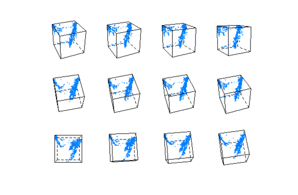Want to share your content on R-bloggers? click here if you have a blog, or here if you don't.

This is the 11th post in a series attempting to recreate the figures in Lattice: Multivariate Data Visualization with R (R code available here) with ggplot2.

Previous parts in this series: Part 1, Part 2, Part 3, Part 4, Part 5, Part 6, Part 7, Part 8, Part 9, Part 10.

## Chapter 11 – Manipulating the “trellis” object

Topics covered:

• Methods for “trellis” objects
• Tukey mean-difference plot
• Other specialized manipulations

### Figure 11.1

 ```> library(lattice) > library(ggplot2)```

lattice

 ```> dp.uspe <- dotplot(t(USPersonalExpenditure), groups = FALSE, + index.cond = function(x, y) median(x), layout = c(1, + 5), type = c("p", "h"), xlab = "Expenditure (billion dollars)") > dp.uspe.log <- dotplot(t(USPersonalExpenditure), groups = FALSE, + index.cond = function(x, y) median(x), layout = c(1, + 5), scales = list(x = list(log = 2)), xlab = "Expenditure (billion dollars)") > plot(dp.uspe, split = c(1, 1, 2, 1), more = TRUE) > plot(dp.uspe.log, split = c(2, 1, 2, 1), more = FALSE)```

ggplot2

 `> library(ggextra)`
 ```> p <- ggplot(melt(USPersonalExpenditure), aes(X2, value, + ymin = 0, ymax = value)) + geom_point() + coord_flip() + + facet_wrap(~X1, ncol = 1) + ylab("Expenditure (billion dollars)") > p1 <- p + geom_linerange() > p2 <- p + scale_y_log2() > print(arrange(p1, p2))```

### Figure 11.2

lattice

 ```> state <- data.frame(state.x77, state.region, state.name) > state\$state.name <- with(state, reorder(reorder(state.name, + Frost), as.numeric(state.region)))```
 ```> dpfrost <- dotplot(state.name ~ Frost | reorder(state.region, + Frost), data = state, layout = c(1, 4), scales = list(y = list(relation = "free"))) > plot(dpfrost, panel.height = list(x = c(16, 13, 9, 12), + unit = "null"))```

ggplot2

 ```> state\$state.region <- with(state, reorder(state.region, + Frost))```
 ```> pg <- ggplot(state, aes(Frost, state.name)) + geom_point() + + facet_grid(state.region ~ ., scales = "free_y", space = "free", + as.table = F) > print(pg)```

### Figure 11.3

lattice

 ```> plot(dpfrost, panel.height = list(x = c(16, 13, 9, 12), + unit = "null")) > print(update(trellis.last.object(), layout = c(1, 1)))```

ggplot2

 ```> pg <- pg %+% subset(state, state.region == "West") + + facet_wrap(~state.region, scales = "free_y") > print(pg)```

### Figure 11.4

lattice

 ```> npanel <- 12 > rot <- list(z = seq(0, 30, length = npanel), x = seq(0, + -80, length = npanel)) > quakeLocs <- cloud(depth ~ long + lat, quakes, pch = ".", + cex = 1.5, panel = function(..., screen) { + pn <- panel.number() + panel.cloud(..., screen = list(z = rot\$z[pn], + x = rot\$x[pn])) + }, xlab = NULL, ylab = NULL, zlab = NULL, scales = list(draw = FALSE), + zlim = c(690, 30), par.settings = list(axis.line = list(col = "transparent"))) > pl <- quakeLocs[rep(1, npanel)] > print(pl)```

ggplot2

 `True 3d not supported in ggplot2.`### Figure 11.5

 `> data(Chem97, package = "mlmRev")`

lattice

 ```> ChemQQ <- qq(gender ~ gcsescore | factor(score), Chem97, + f.value = ppoints(100), strip = strip.custom(style = 5)) > pl <- tmd(ChemQQ) > print(pl)```

ggplot2

 ```> q <- function(x, probs = ppoints(100)) { + data.frame(q = probs, value = quantile(x, probs)) + } > Chem97.q <- ddply(Chem97, c("gender", "score"), function(df) q(df\$gcsescore)) > Chem97.df <- recast(Chem97.q, score + q ~ gender, id.var = 1:3)```
 ```> pg <- ggplot(Chem97.df, aes(M, F)) + geom_point(aes(x = (M + + F)/2, y = F - M)) + facet_wrap(~score) + xlab("mean") + + ylab("difference") > print(pg)```

### Figure 11.6

 ```> library("latticeExtra") > data(biocAccess)```

lattice

 ```> baxy <- xyplot(log10(counts) ~ hour | month + weekday, + biocAccess, type = c("p", "a"), as.table = TRUE, + pch = ".", cex = 2, col.line = "black") > dimnames(baxy)\$month <- month.name[1:5] > pl <- useOuterStrips(baxy) > print(pl)```

ggplot2

 ```> pg <- ggplot(biocAccess, aes(hour, log10(counts))) + + geom_point(colour = "steelblue", size = 1) + geom_line(stat = "summary", + fun.y = mean) + facet_grid(weekday ~ month) > print(pg)```